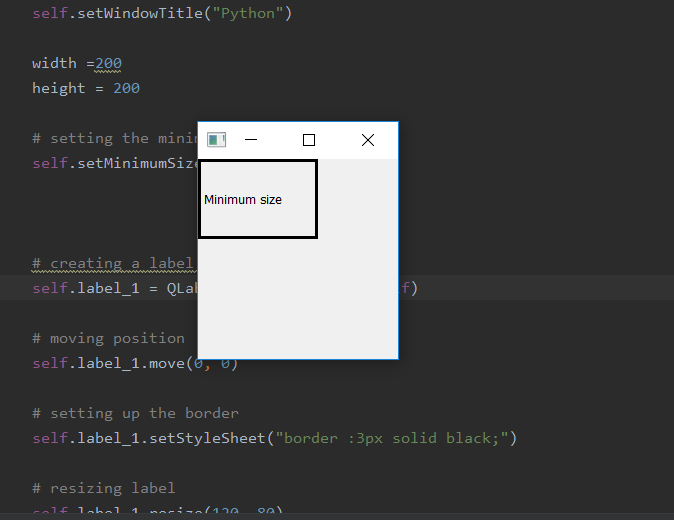GeeksforGeeks App
Open AppBrowser
Continue

# PyQt5 – How to set minimum size of window | setMinimumSize method

We know that we can resize the window of PyQt5 application, but while shrinking the window size we can set minimum size so that it can’t be shrank any more. In this article, we will see how to do this.

In order to do this we will use `setMinimumSize()` method.

Syntax : self.setMinimumSize(width, height)

Argument : It takes two integer as argument.

Action performed : It sets the minimum size of window.

Code :

 `# importing the required libraries`` ` `from` `PyQt5.QtCore ``import` `*` `from` `PyQt5.QtGui ``import` `*` `from` `PyQt5.QtWidgets ``import` `*` `import` `sys`` ` ` ` `class` `Window(QMainWindow):``    ``def` `__init__(``self``):``        ``super``().__init__()`` ` ` ` `        ``# set the title``        ``self``.setWindowTitle(``"Python"``)`` ` `        ``width ``=` `200``        ``height ``=` `200`` ` `        ``# setting the minimum size``        ``self``.setMinimumSize(width, height)``         ` `        ``# creating a label widget``        ``self``.label_1 ``=` `QLabel(``"Minimum size"``, ``self``)`` ` `        ``# moving position``        ``self``.label_1.move(``0``, ``0``)`` ` `        ``# setting up the border``        ``self``.label_1.setStyleSheet(``"border :3px solid black;"``)`` ` `        ``# resizing label``        ``self``.label_1.resize(``120``, ``80``)`` ` `        ``# show all the widgets``        ``self``.show()`` ` ` ` `# create pyqt5 app``App ``=` `QApplication(sys.argv)`` ` `# create the instance of our Window``window ``=` `Window()`` ` `# start the app``sys.exit(App.``exec``())`

Output :My Personal Notes arrow_drop_up